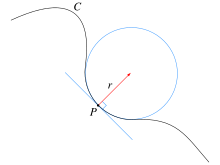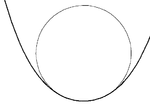# Osculating circle

Osculating circleAn osculating circle

In differential geometry of curves, the osculating circle of a sufficiently smooth plane curve at a given point p on the curve has been traditionally defined as the circle passing through p and a pair of additional points on the curve infinitesimally close to p. Its center lies on the inner normal line, and its curvature is the same as that of the given curve at that point. This circle, which is the one among all tangent circles at the given point that approaches the curve most tightly, was named circulus osculans (Latin for "kissing circle") by Leibniz.

The center and radius of the osculating circle at a given point are called center of curvature and radius of curvature of the curve at that point. A geometric construction was described by Isaac Newton in his Principia:

There being given, in any places, the velocity with which a body describes a given figure, by means of forces directed to some common centre: to find that centre.

Isaac Newton, Principia; PROPOSITION V. PROBLEM I.

## Description in lay terms

Imagine a car moving along a curved road on a vast flat plane. Suddenly, at one point along the road, the steering wheel locks in its present position. Thereafter, the car moves in a circle that "kisses" the road at the point of locking. The curvature of the circle is equal to that of the road at that point. That circle is the osculating circle of the road curve at that point.

## Mathematical description

Let γ(s) be a regular parametric curve, where s is the arc length, or natural parameter. This determines the unit tangent vector T, the unit normal vector N, the signed curvature k(s) and the radius of curvature at each point:$T(s)=\gamma'(s),\quad T'(s)=k(s)N(s),\quad R(s)=\frac{1}{\left|k(s)\right|}.$

Suppose that P is a point on C where k ≠ 0. The corresponding center of curvature is the point Q at distance R along N, in the same direction if k is positive and in the opposite direction if k is negative. The circle with center at Q and with radius R is called the osculating circle to the curve C at the point P.

If C is a regular space curve then the osculating circle is defined in a similar way, using the principal normal vector N. It lies in the osculating plane, the plane spanned by the tangent and principal normal vectors T and N at the point P.

## Properties

For a curve C given by a sufficiently smooth parametric equations (twice continuously differentiable), the osculating circle may be obtained by a limiting procedure: it is the limit of the circles passing through three distinct points on C as these points approach P. This is entirely analogous to the construction of the tangent to a curve as a limit of the secant lines through pairs of distinct points on C approaching P.

The osculating circle S to a plane curve C at a regular point P can be characterized by the following properties:

• The circle S passes through P.
• The circle S and the curve C have the common tangent line at P, and therefore the common normal line.
• Close to P, the distance between the points of the curve C and the circle S in the normal direction decays as the cube or a higher power of the distance to P in the tangential direction.

This is usually expressed as "the curve and its osculating circle have the third or higher order contact" at P. Loosely speaking, the vector functions representing C and S agree together with their first and second derivatives at P.A circle with fourth order contact at a vertex of a curve

If the derivative of the curvature with respect to s is nonzero at P then the osculating circle crosses the curve C at P. Points P at which the derivative of the curvature is zero are called vertices. If P is a vertex then C and its osculating circle have contact of order at least four. If, moreover, the curvature has a non-zero local maximum or minimum at P then the osculating circle touches the curve C at P but does not cross it.

The curve C may be obtained as the envelope of the one-parameter family of its osculating circles. Their centers, i.e. the centers of curvature, form another curve, called the evolute of C. Vertices of C correspond to singular points on its evolute.

## References and notes

1. ^ Actually, point P plus two additional points, one on either side of P will do. See Lamb (on line): Horace Lamb (1897). An Elementary Course of Infinitesimal Calculus. University Press. p. 406.